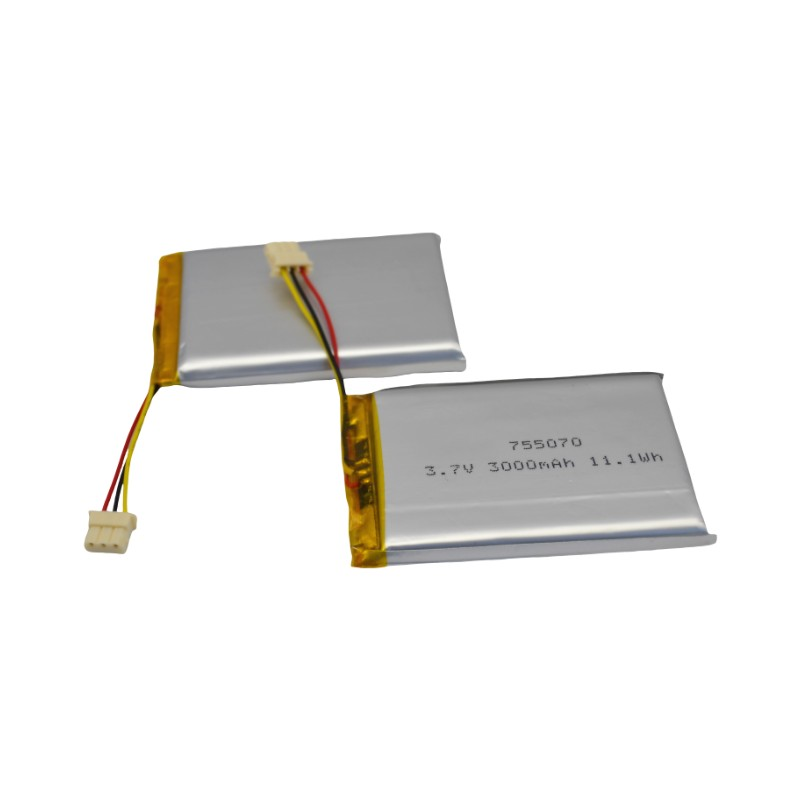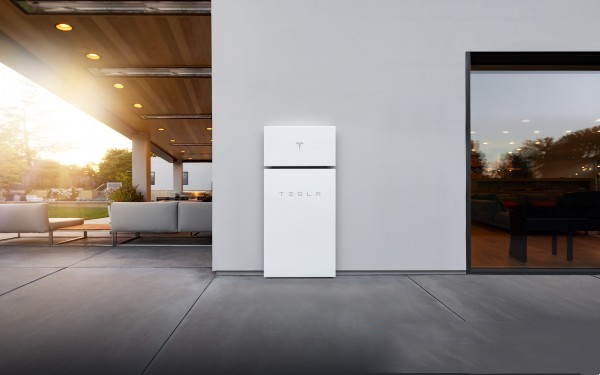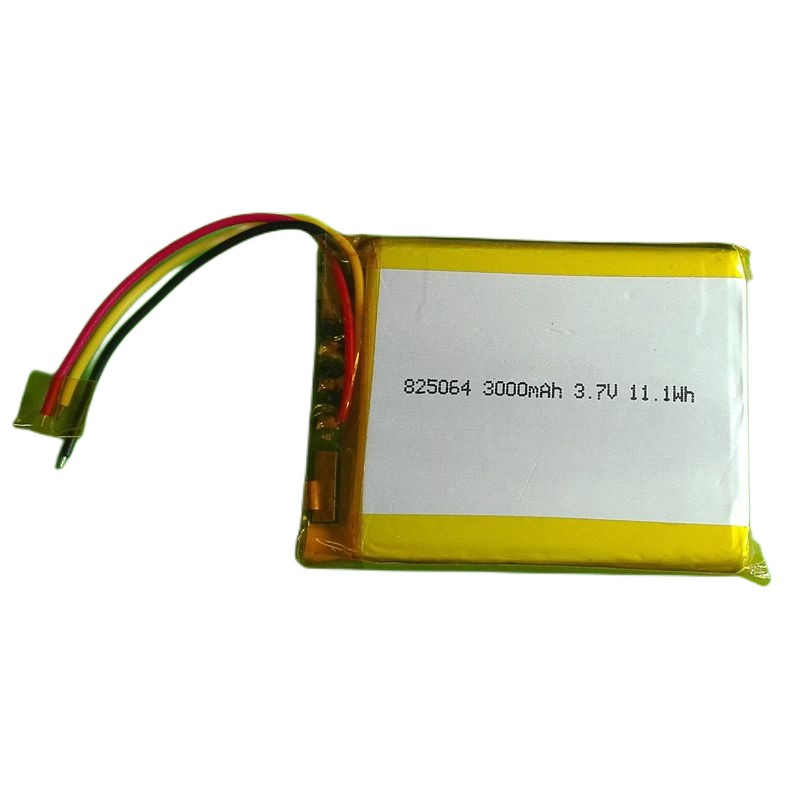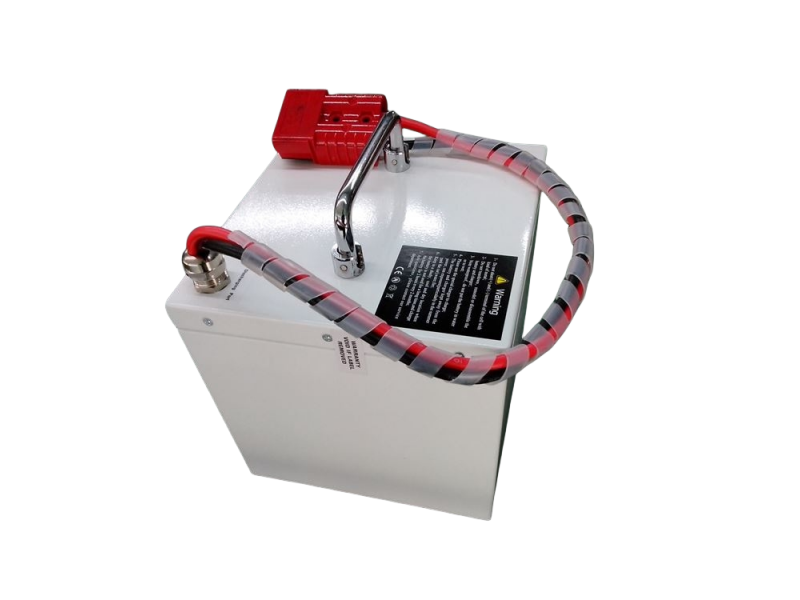# How to configure the home solar power generation system?

Views : 128
Author : topwellpower
Update time : 2023-05-31 17:54:34
Today, Yichun Topwell Power Co., Ltd will show you how to calculate the data of some common solar power generation systems, and use this to choose suitable accessories.
First of all, we need to understand some basic circuit knowledge, such as Ohm's law (that is, voltage = current * resistance), and the calculation formula about power:
Current (A) x Voltage (V) = Power (W)
Current × power consumption time = battery capacity Ah
Simply understand that 1AH is 1A discharge for 1 hour
After having these knowledge backgrounds, we also need to know some conditions:
2. Working hours per day
3. Use (installation) location
4. Sunshine time
Example 1: Assume that the required load requirement is 48W12V, and it works 10 hours a day. If it is necessary to ensure that the load can still work normally for 3 consecutive cloudy days.
How to match the solar system? (Place of use: Fujian, 4 hours of standard sunshine)
calculation process:
⑴ Calculate the power of solar photovoltaic panels:
The daily power consumption of the load is: 48W*10H=480WH
480WH ÷ 4H =120W
(18v solar panels charge 12v batteries, 36v solar panels charge 24v batteries)
Because the solar panel is not only used for the load, but also to charge the battery, so the solar panel must be greater than 120W.
⑵Battery capacity calculation:
The power consumed by the load for three days is 480WH*3D=1440WH
1440WH÷12V=120AH
Because the choice of battery needs to leave 20% of the balance
Therefore, the battery capacity is: 120AH*1.2=144AH
So the system matching is:
Solar panel voltage: 18V
Solar panel power: 120W or more
Battery: 12V144AH
Example 2: How many hours can a 100W solar panel generate electricity for a 40W/12V LED light in a day? If the LED lights work for 8 hours a day to ensure continuous work in rainy days for 3 days, how big should the battery be? (Installation location: Fujian, the sunshine time is calculated according to 4 hours)
calculation process:
(1) The electricity generated by solar energy in one day is: 100W*4H=400WH
It is known that the power of the LED lamp is 40W
400WH/40W=10H
Therefore, the LED light can work for 10H
(2) It is known that the LED lamp works 8H per day, so the power consumption of the LED lamp per day is 40W*8H=320WH
The power consumption for 3 days is 3D*320WH=960WH
960WH/12V=80AH
80AH*1.2=96AH
So choose 12V96AH battery
Example 3: Given that the battery voltage is 12V and the capacity is 200AH, how many solar panels do you need to charge the battery in two days? (Daily average effective light is 4H)
calculation process:
(1) The power stored in the battery is 12V*200AH=2400WH
2400WH/4H=600W
600W/2=300W
Therefore, a 18V300W solar panel is required.
I hope that these few simple examples can help you. If you have any purchase needs for solar power generation systems, you can also contact [email protected] to learn more.
Related NewsLithium Polymer Battery: Benefits and ApplicationsUnderstanding the Cost of Installing a Tesla Powerwall Battery System for Home Energy StorageTOPWELL-lithium polymer battery and 18650 Li-ion Battery products videoAdvancements in Lithium-Ion Battery Technology Propel Electric Vehicle Industry Forward